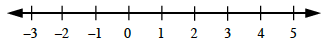### Home > CC1MN > Chapter 3 > Lesson 3.2.4 > Problem3-128

3-128.

Evaluate each absolute value expression below.

Absolute value means distance away from zero on a number line.

Consult the number line below. How far is each value from $0$?1. $| 2 | + | 4 |$

Since $2$ and $4$ are already positive, just add.

2. $| - 3 | + | 5 |$

How far is $-3$ from $0$?

$\left|−3\right| = 3$

$\left|5\right| =5$

$3 + 5 = 8$

3. $| - 7 | + | - 1 |$

4. $- | 6 |$

$−6$